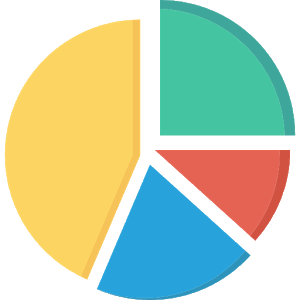\$3.29

# Android Giveaway of the Day - Fraction calculator with steps

We present to your attention a calculator for fractions!
\$3.29 期限切れ
ユーザーの評価: 0

このgiveawayオファーはすでに期限が切れています。Fraction calculator with stepsが今紹介されています。

We present to your attention a calculator for fractions! A very useful and convenient application for solving everyday tasks. The calculator for fractions produces addition, subtraction, multiplication and division of ordinary fractions, mixed fractions and integers. Has a sound and vibration feedback. The progress of the solution is divided into separate actions and is displayed during the calculation of the example. With this program, checking homework on mathematics will be for you an exciting activity!

_D&J_

Education

2.01

2.7M

Everyone

4.0.3 and up

## コメント Fraction calculator with steps

Thank you for voting!